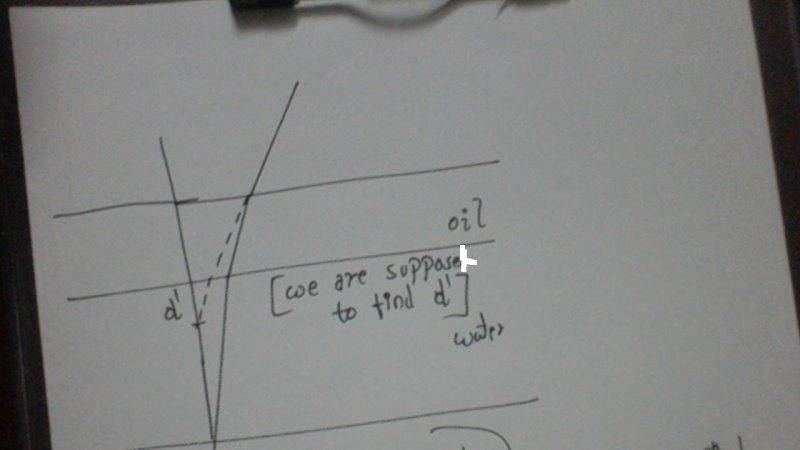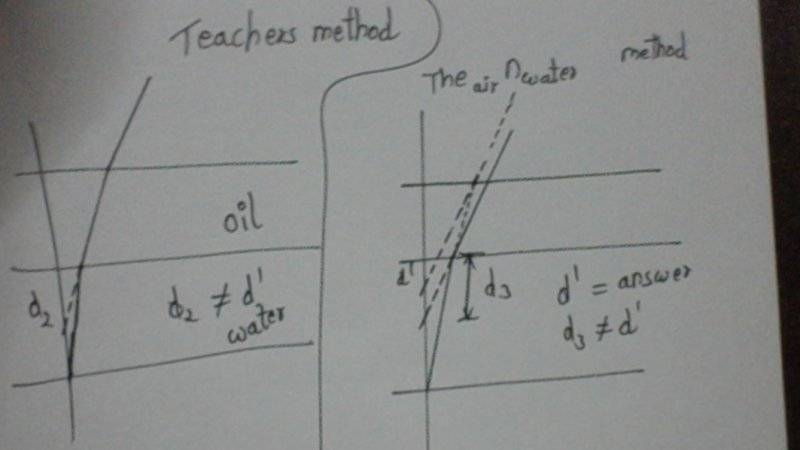# Normal shift

Gold Member

## Homework Statement

This is how my teacher dictated the question...
---A layer of oil 3 cm thick is floating on a layer of coloured water 5 cm thick, the RI of the coloured water with respect to the oil is ##\frac{5}{3}##. The apparent depth of the liquid is ##\frac{36}{7} cm##. What is the RI of oil(with respect to air).

## Homework Equations

##d' = \frac{d}{n}##
n is RI

## The Attempt at a Solution

This is what my teacher told us to do, after we tried to do it.
$$\frac{36}{7} = \frac{d_{oil}}{n_{oil ~w.r.t~ air}} + \frac{d_{water}}{ n_{water~ w.r.t~ oil}}$$
solving this we get ##n_{oil} = \frac{7}{5}##
I have a feeling this is wrong, though my teacher says it is right.
Particularly in the place where we use ##n_{water ~wrt~ oil}## wouldn't this actually mean that the level of water is being viewed from the oíl. Which is not the case.
I thought maybe if we took ##n_{water ~wrt~ air}## we might get the answer but i'm starting to think., even that won't give me the answer.
could you point me in the right direction..
thank you

...wouldn't this actually mean that the level of water is being viewed from the oíl. Which is not the case.
But isn't it the case?

haruspex
Homework Helper
Gold Member
2020 Award

## Homework Statement

This is how my teacher dictated the question...
---A layer of oil 3 cm thick is floating on a layer of coloured water 5 cm thick, the RI of the coloured water with respect to the oil is ##\frac{5}{3}##. The apparent depth of the liquid is ##\frac{36}{7} cm##. What is the RI of oil(with respect to air).

## Homework Equations

##d' = \frac{d}{n}##
n is RI

## The Attempt at a Solution

This is what my teacher told us to do, after we tried to do it.
$$\frac{36}{7} = \frac{d_{oil}}{n_{oil ~w.r.t~ air}} + \frac{d_{water}}{ n_{water~ w.r.t~ oil}}$$
solving this we get ##n_{oil} = \frac{7}{5}##
I have a feeling this is wrong, though my teacher says it is right.
Particularly in the place where we use ##n_{water ~wrt~ oil}## wouldn't this actually mean that the level of water is being viewed from the oíl. Which is not the case.
I thought maybe if we took ##n_{water ~wrt~ air}## we might get the answer but i'm starting to think., even that won't give me the answer.
could you point me in the right direction..
thank you
Your teacher is wrong, and your suggestion is correct (why do you think that is wrong too?)

Gold Member
and your suggestion is correct (why do you think that is wrong too?)
It was more of a guess.. well look at this...View attachment 81681so the d₃ we find is not d'..
d₃ is different from d₂because we are considering RI of water wrt air.. and hence the refracted ray goes in a different path..
Is this argument valid??

Last edited:
Gold Member
But isn't it the case?
no its being viewed from the air through the oil..

haruspex
Homework Helper
Gold Member
2020 Award
and hence the refracted ray goes in a different path..
Is this argument valid??
I haven't tried to follow all that, but it's easy to show the teacher's answer is wrong. Consider what happens as the depth of oil tends to zero. According to your teacher's answer an infinitesimal layer of oil radically alters the apparent depth.

To get the right answer, work in terms of images. Viewed from within the oil, just below the air boundary, the image is at depth doil+dwater/noil-water. Multiply that appropriately to get the apparent depth of that image when viewed from just above the boundary.

Gold Member
I think i get the idea, if i try considering the oil surface, the equation reduces to the same equation we get when we directly use the ##n_{water~wrt~air}##
Thank you..

•Rohit H
Come to class, I will teach you !!!

Gold Member
Come to class, I will teach you !!!
See post #7